• 视频教程• 秒刷功能• 编辑器快捷键
• 关注按钮
• 添加背景图
• 弹幕文字修改增删• 时间轴增加节点
• DIY收藏，一键套用
• 图片快传• 二维码
• 导入公众号# 要提升微信看一看推荐混排的长期收益？试试深度强化学习

（1）基本概念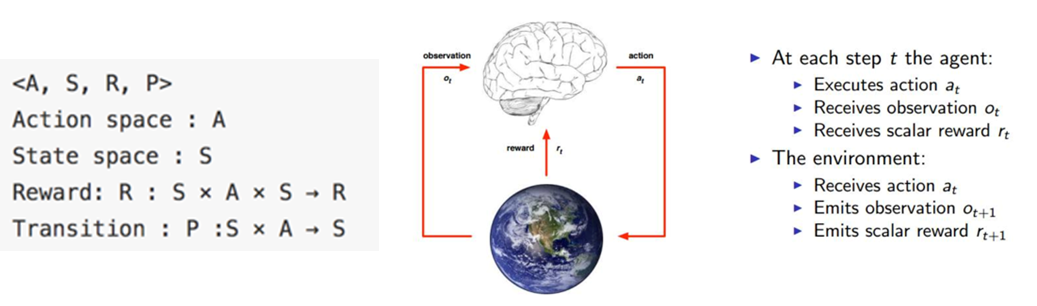（2）与监督学习，非监督学习的区别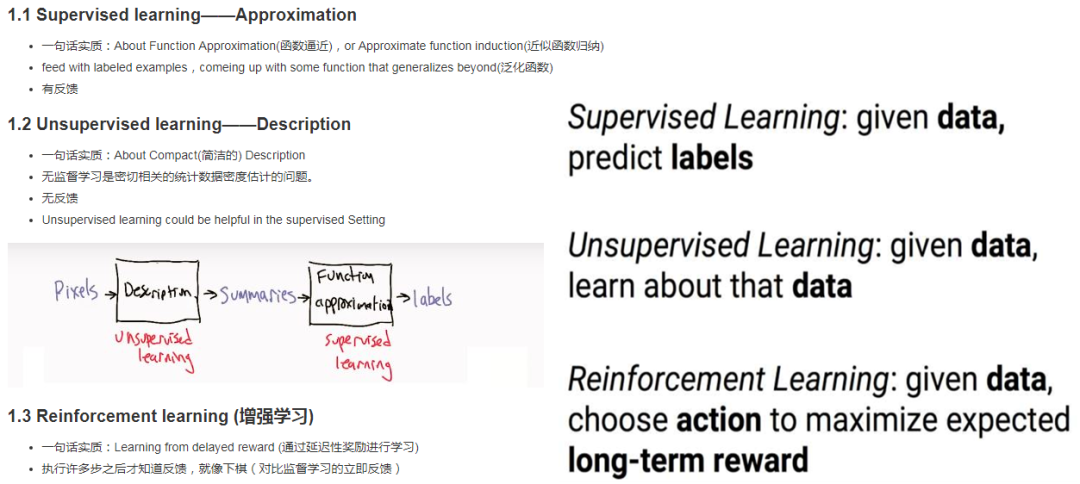（3）Multi-armed bandit 多臂赌博机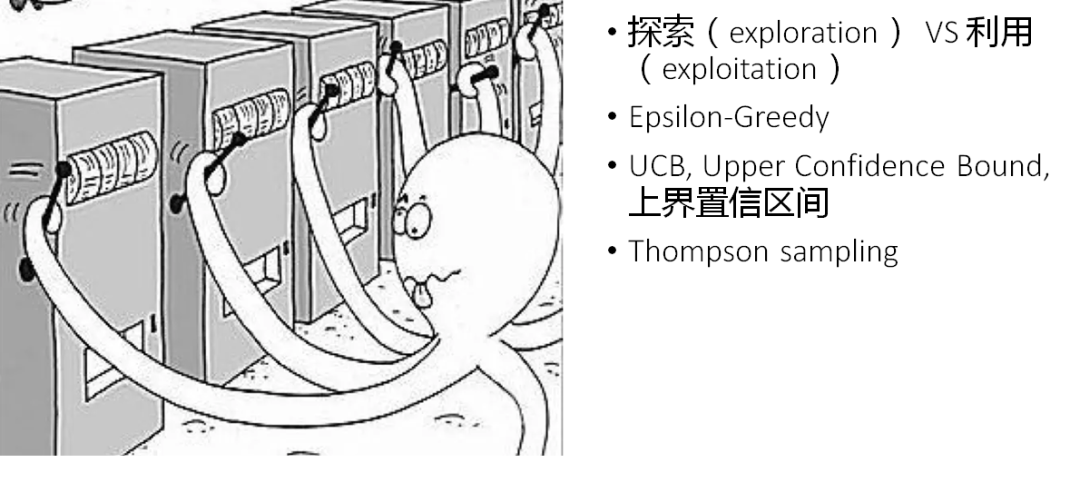（4）强化学习的算法和AlphaGo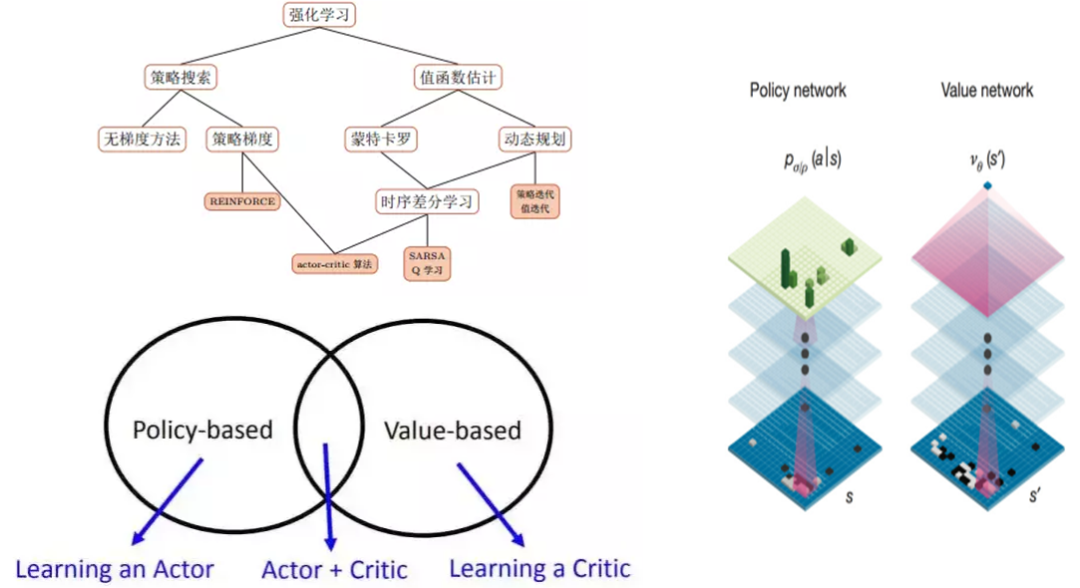（5）强化学习实践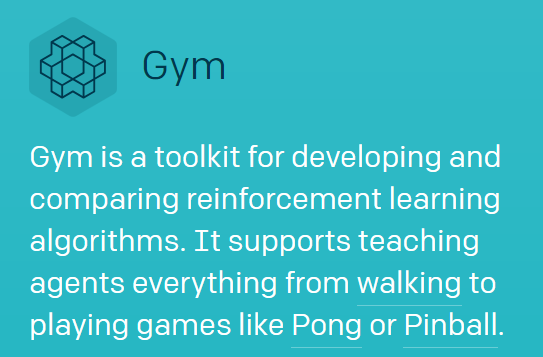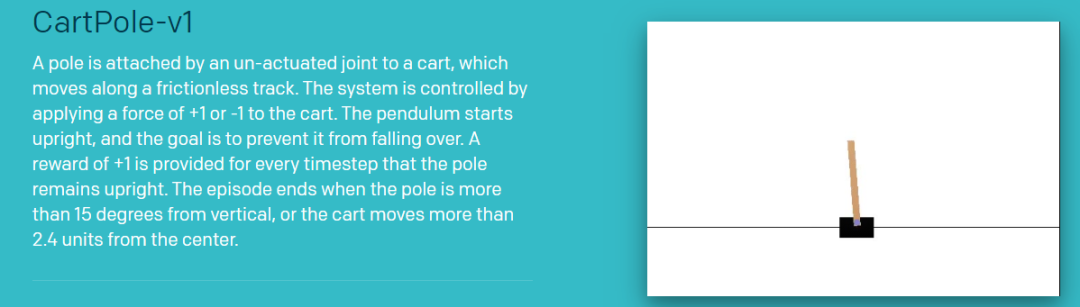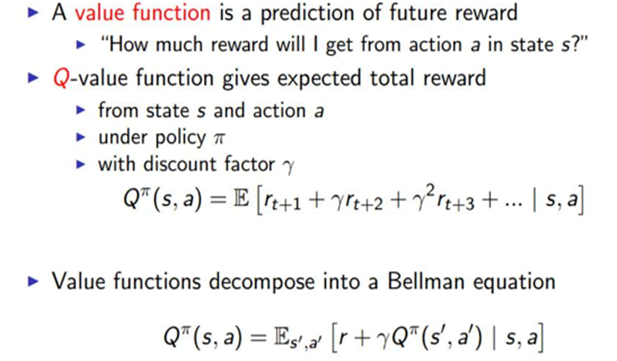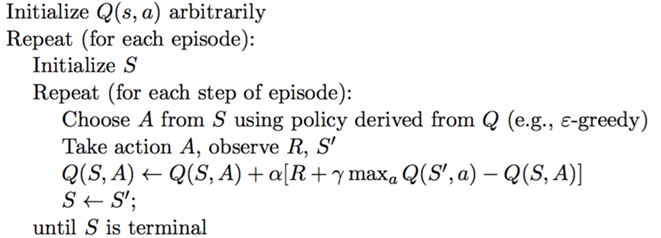import gym

import random

import numpy

N_BINS = [5, 5, 5, 5]

LEARNING_RATE=0.05

DISCOUNT_FACTOR=0.9

EPS = 0.3

MIN_VALUES = [-0.5,-2.0,-0.5,-3.0]

MAX_VALUES = [0.5,2.0,0.5,3.0]

BINS = [numpy.linspace(MIN_VALUES[i], MAX_VALUES[i], N_BINS[i]) for i in xrange(4)]

def discretize(obs):

return tuple([int(numpy.digitize(obs[i], BINS[i])) for i in xrange(4)])

qv = {}

env = gym.make('CartPole-v0')

print(env.action_space)

print(env.observation_space)

an = env.action_space.n

def get(s, a):

global qv

if (s, a) not in qv:

return 0

return qv[(s, a)]

def update(s, a, s1, r):

global qv

nows = get(s, a)

m0 = get(s1, 0)

m1 = get(s1, 1)

if m0 < m1:

m0 = m1

qv[(s, a)] = nows + LEARNING_RATE * (r + DISCOUNT_FACTOR * m0 - nows)

for i in range(500000):

obs = env.reset()

if i % 1000 == 0:

print i

for _ in range(5000):

s = discretize(obs)

s_0 = get(s, 0)

nowa = 0

s_1 = get(s, 1)

if s_1 > s_0:

nowa = 1

if random.random() <= EPS:

nowa = 1 - nowa

obs, reward, done, info = env.step(nowa)

s1 = discretize(obs)

if done:

reward = -10

update(s, nowa, s1, reward)

if done:

break

for i_episode in range(1):

obs = env.reset()

for t in range(5000):

env.render()

s = discretize(obs)

maxs = get(s, 0)

maxa = 0

nows = get(s, 1)

if nows > maxs:

maxa = 1

obs, reward, done, info = env.step(maxa)

if done:

print("Episode finished after {} timesteps".format(t+1))

break

（1）看一看混排（2）统一的点击率预估排序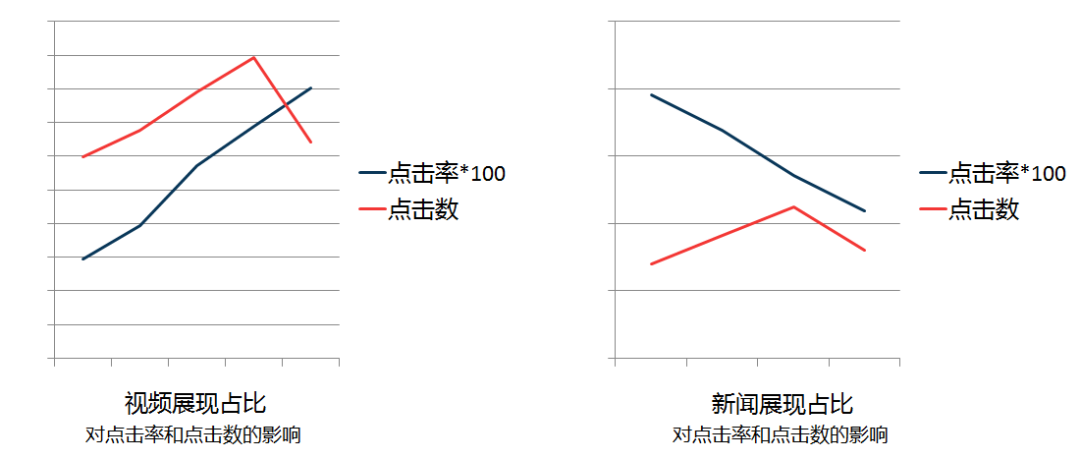（3）强化学习的引入 - 优化长期收益

Agent是我们的推荐系统，Action是我们推荐了什么内容，Reward是反馈信息，包括点击、负反馈、退出等。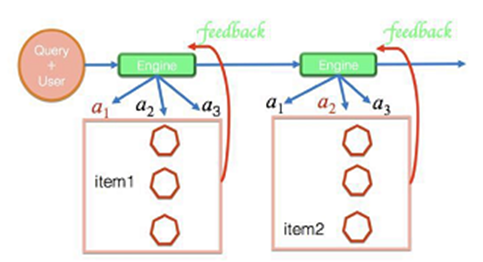（4）强化学习的优势

Case

mp，video，video（0，1，1）

video，mp，mp（1，0，0）

video，video，video（1，0，0）

（1）Session wise recommendation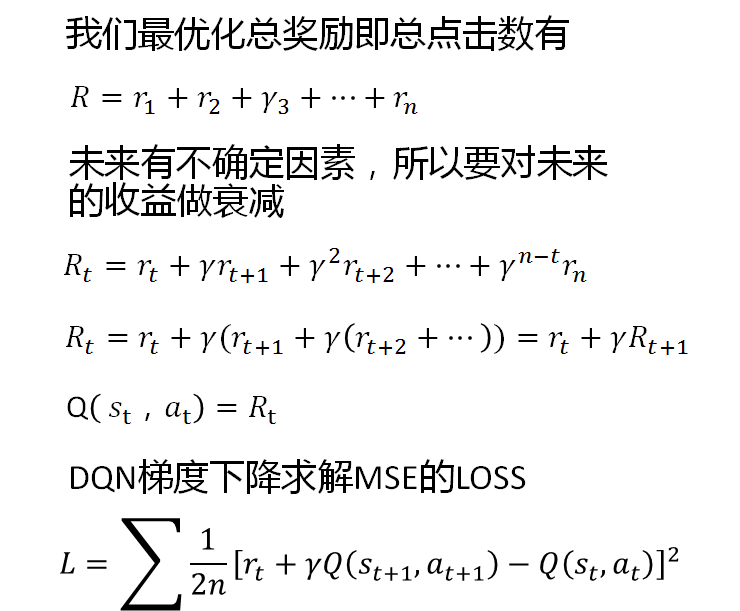（2）Personal DQN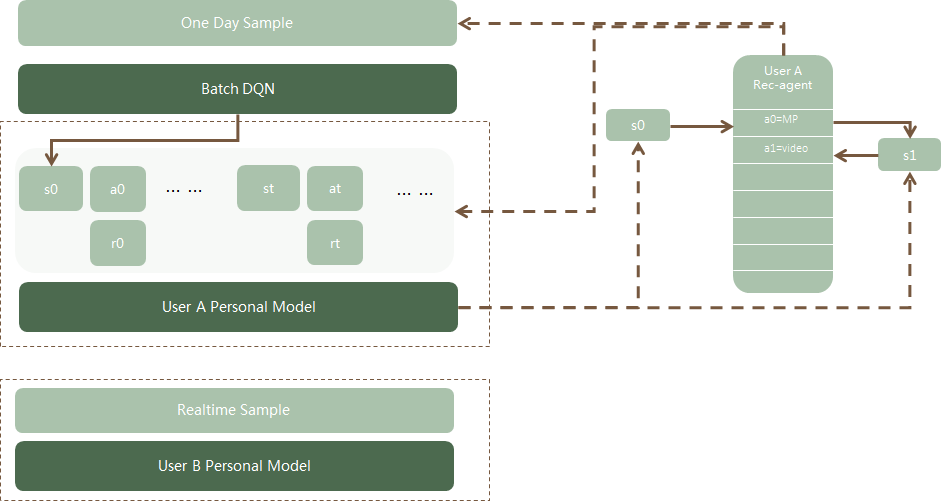（3）离线评估 AUC？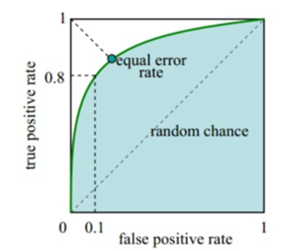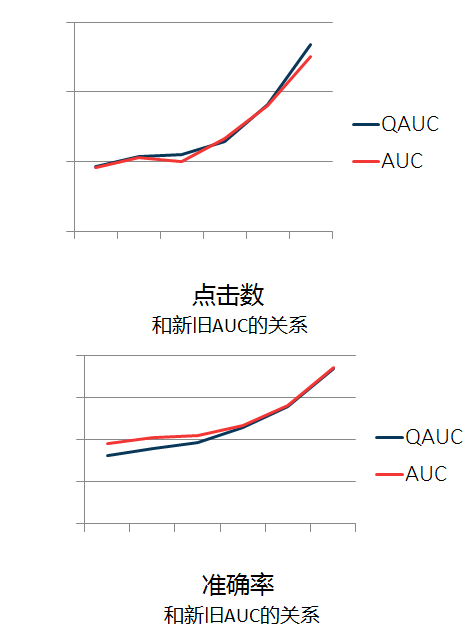（4）线上效果

（5）模型优化

Session based recommendation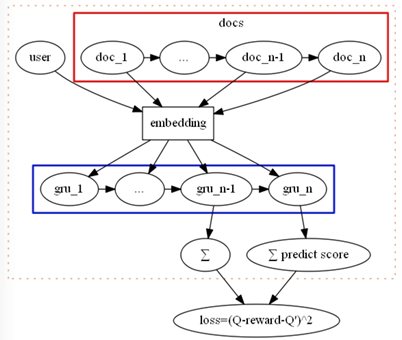（6）模型优化

Bloom embedding & Dueling DQN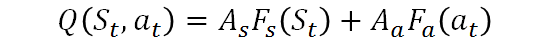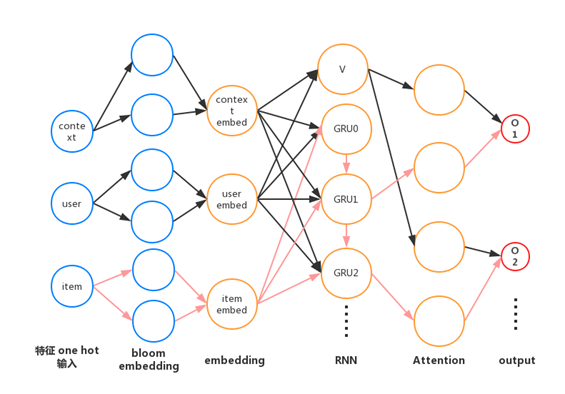Bloom embedding用于减小hash之间的冲突利用 Dueling DQN的方式，将user context的信息抽离出来，最后利用attention的方式连接，能够让模型很好地学到时间、位置等bias信息。

（7）模型优化Double DQN &

Dueling Double DQN (aka DDDQN)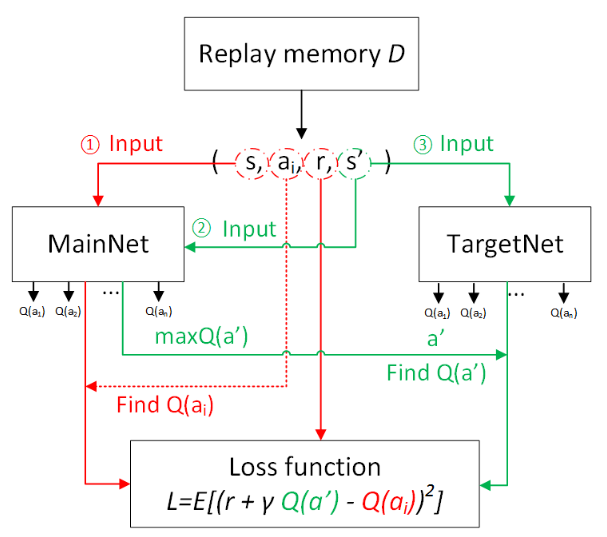（8）负反馈 Reward & Focal loss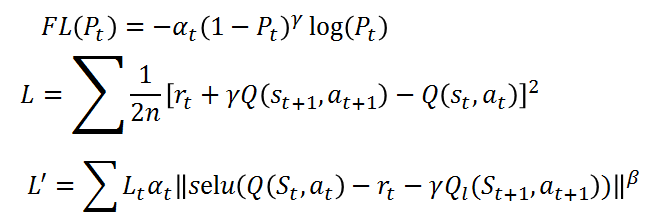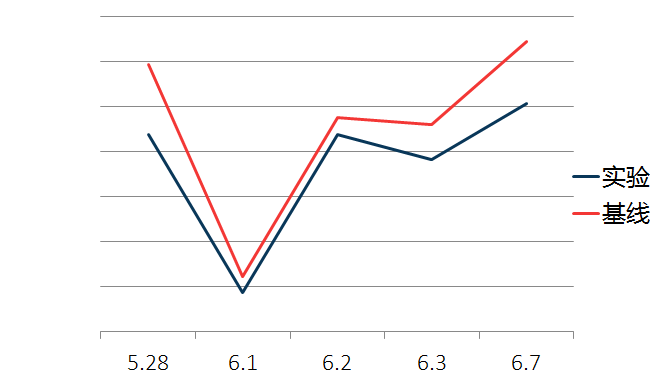AC 和 GAN

Ian Goodfellow（生成对抗网络之父）